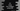# C# program to swap two numbers using XOR## How to swap two numbers using XOR in C#:

XOR operator can be used to swap two numbers. This is a bitwise operation and it converts the number to binary and performs XOR on each digit of both numbers.

In C#, XOR is denoted by ^.

The below algorithm is used to swap two numbers using XOR:

``````first = first ^ second
second = first ^ second
first = first ^ second``````

Where first and second are the first and second numbers.

We can write a C# program that will take both numbers as input from the user and swap them using XOR.

### C# program:

Below is the complete C# program:

``````using System;

public class Program
{
public static void Main()
{
int first,second;

Console.WriteLine("Enter the first number : ");

Console.WriteLine("Enter the second character : ");

Console.WriteLine("Before swapping first = {0}, second = {1}",first,second);

first = first ^ second;
second = first ^ second;
first = first ^ second;

Console.WriteLine("After swapping first = {0}, second = {1}",first,second);
}
}``````

Here,

• first and second are two integer variables to hold the user input values.
• It is reading these numbers as input from the user.
• It prints the numbers before and after swapping is done.
• For swapping, it uses XOR similar to the algorithm we discussed above.

### Sample output:

If you run this program, it will give output as like below:

``````Enter the first number :
10
Enter the second character :
11
Before swapping first = 10, second = 11
After swapping first = 11, second = 10``````

As you can see here, the entered numbers are swapped.